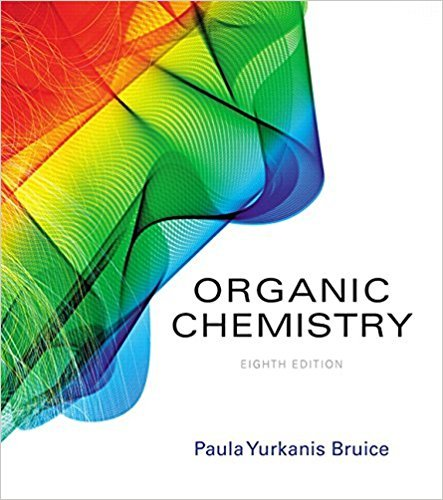×
×

# Solutions for Chapter 20: The Organic Chemistry of Carbohydrates## Full solutions for Organic Chemistry | 8th Edition

ISBN: 9780134042282Solutions for Chapter 20: The Organic Chemistry of Carbohydrates

Solutions for Chapter 20
4 5 0 409 Reviews
18
3
##### ISBN: 9780134042282

Since 73 problems in chapter 20: The Organic Chemistry of Carbohydrates have been answered, more than 31227 students have viewed full step-by-step solutions from this chapter. Chapter 20: The Organic Chemistry of Carbohydrates includes 73 full step-by-step solutions. This expansive textbook survival guide covers the following chapters and their solutions. This textbook survival guide was created for the textbook: Organic Chemistry, edition: 8. Organic Chemistry was written by and is associated to the ISBN: 9780134042282.

Key Chemistry Terms and definitions covered in this textbook
• absorption spectrum

A pattern of variation in the amount of light absorbed by a sample as a function of wavelength. (Section 23.5)

• Allene

The compound CH2"C"CH2. Any compound that contains adjacent carbon-carbon double bonds; that is, any molecule that contains a C"C"C functional group.

• Brønsted-Lowry base

A proton acceptor

• catalyst

A compound that can speed up the rate of a reaction without itself being consumed by the reaction.

• chemical equation

A representation of a chemical reaction using the chemical formulas of the reactants and products; a balanced chemical equation contains equal numbers of atoms of each element on both sides of the equation. (Section 3.1)

• chemical equation.

An equation that uses chemical symbols to show what happens during a chemical reaction. (3.7)

A radical process in which two radicals join together and form a bond.

• degree of unsaturation

The absence of two hydrogen atoms associated with a ring or a p bond.

• dispersion forces

Intermolecular forces resulting from attractions between induced dipoles. Also called London dispersion forces. (Section 11.2)

• formation constant

For a metal ion complex, the equilibrium constant for formation of the complex from the metal ion and base species present in solution. It is a measure of the tendency of the complex to form. (Section 17.5)

• hydrophobic

A nonpolar group that does not have favorable interactions with water.

• Ligand

A Lewis base bonded to a metal atom in a coordination compound. It may bond strongly or weakly.

• metallic hydrides

Compounds formed when hydrogen reacts with transition metals; these compounds contain the hydride ion, H-. (Section 22.2)

• microstate

The state of a system at a particular instant; one of many possible energetically equivalent ways to arrange the components of a system to achieve a particular state. (Section 19.3)

• photodissociation

The breaking of a molecule into two or more neutral fragments as a result of absorption of light. (Section 18.2)

• Ring current

An applied magnetic fi eld causes the p electrons of an aromatic ring to circulate, giving rise to the so-called ring current and an associated magnetic fi eld that opposes the applied fi eld in the middle of the ring but reinforces the applied fi eld on the outside of the ring.

• shielded

In NMR spectroscopy,protons or carbon atoms whose surrounding electron density is rich.

• Signal

A recording in an NMR spectrum of a nuclear magnetic resonance

• spin-pairing energy

The energy required to pair an electron with another electron occupying an orbital. (Section 23.6)

• Tollens’ reagent

A solution prepared by dissolving Ag2O in aqueous ammonia; used for selective oxidation of an aldehyde to a carboxylic acid.

×